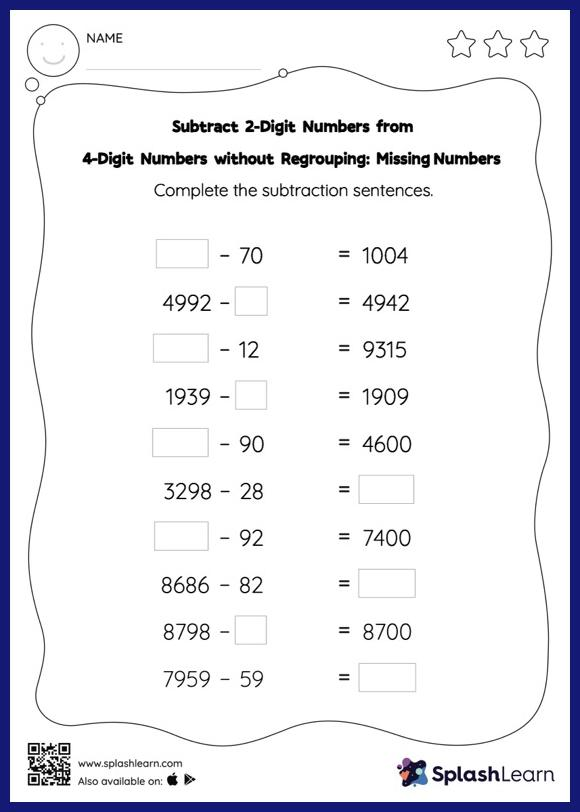# Subtract 2-Digit Numbers from 4-Digit Numbers without Regrouping: Missing Numbers Worksheet

Home > Subtract 2-Digit Numbers from 4-Digit Numbers without Regrouping: Missing NumbersThis worksheet strengthens students' accuracy and strategic flexibility while they subtract 2-digit numbers from 4-digit numbers without regrouping. Students use the count back strategy or the relationship between addition and subtraction to find the missing number in this worksheet. However, they do not need to regroup numbers to get to the answer in subtract 2-digit numbers from 4-digit numbers without regrouping worksheet.